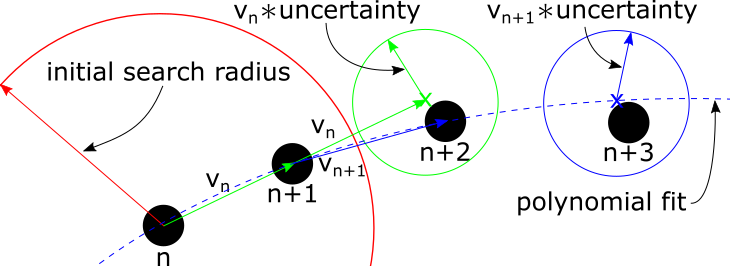# Track Objects¶

Now that the frame is processed and objects identified, the objects can be tracked by selecting a tracking method from the Method drop-down list.## Poly Projection¶

The poly projection algorithm is a projection based approach to finding the same object across successive frames. By fitting the past particle locations with a polynomial, the polynomial can be evaluated to predict where the particle location should be. For details of the algorithm see Poly Projection.This first step in optimizing the tracking routine is to select appropriate parameters affect the search radius. First, un-select the Filter tracks check-box to see all the objects that are being tracked. Next, play with the following three parameters, while moving forward and backward through the first couple frames:

1. Initial Search Radius - The initial search radius is used to find the closest object at $$n+1$$. This radius should be just large enough to capture the fastest moving object.

2. Uncertainty - The uncertainty is a multiplier on the velocity used to grow or shrink the the subsequent search radius. This lets the search radius change with changing velocities.

3. Minimum Search Radius - The minimum search radius is used to prevent the subsequent search radius from getting to small. This is need to make sure that objects that stop moving are still tracked because using a search radius of $$r_{search}(n) = uncertainty*v(n-1)$$ could end up being zero if the velocity is zero.

Note

To see the search radius, go the visualization tab and select the Show search check-box.

After the search radius have been optimized, move on to the polynomial settings. Generally leaving the settings is fine. If the objects have little acceleration and are moving linearly, turning off the polynomial by un-selecting the Use polynomial check-box can increase the speed because the algorithm avoids using a least-squares regression to fit the polynomials.

Finally, turn on the track filtering by selecting the Filter tracks check-box. Setting a realistic maximum velocity and increasing the number of consecutive points in the track can help reduce tracks connecting the wrong particles.